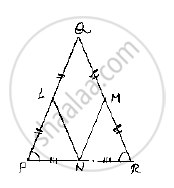Share

Books Shortlist

# In a δPqr, If Pq = Qr and L, M and N Are the Mid-points of the Sides Pq, Qr and Rp Respectively. Prove That: Ln = Mn. - CBSE Class 9 - Mathematics

ConceptCongruence of Triangles

#### Question

In a ΔPQR, if PQ = QR and L, M and N are the mid-points of the sides PQ, QR and RP
respectively. Prove that: LN = MN.

#### Solution

Given that, in PQR, PQ QRand L,M,N are midpoints of the sides PQ, QP and RP
respectively and given to prove that LN  MN
Here we can observe that PQR is and isosceles triangle
⇒PQ =QR and ∠QPR =∠QRP ……..(1)
And also, L and M are midpoints of PQ and QR respectively
⇒ PL=LQ=(PQ)/2,QM=MR=(QR)/2

And also, PQ=QR

⇒ PL=LQ=QM=MR=(PQ)/2=(QR)/2 .............(2)

Now, consider ΔLPN  and ,Δ MRN
LP= MR           [From – (2)]
∠LPN =∠MRN   [From – (1)]
∵∠QPR  and ∠LPN  and ∠ QRP  and ∠MRN are same
PN= NR  [∵N is midpoint of PR]
So, by SAS congruence criterion, we have LPN≅  MRN
⇒LN =MN
[ ∵Corresponding parts of congruent triangles are equal]Is there an error in this question or solution?

#### APPEARS IN

Solution In a δPqr, If Pq = Qr and L, M and N Are the Mid-points of the Sides Pq, Qr and Rp Respectively. Prove That: Ln = Mn. Concept: Congruence of Triangles.
S